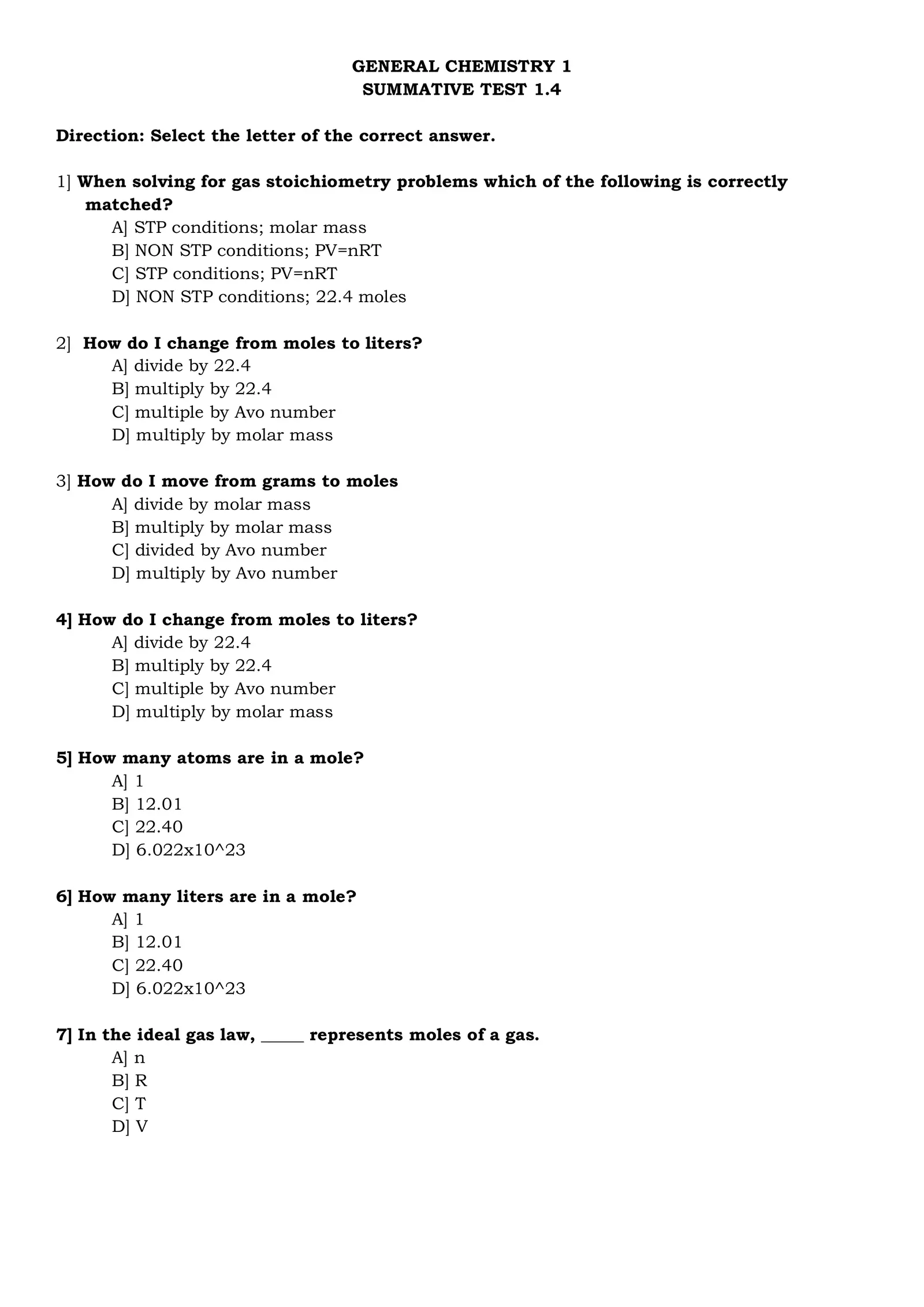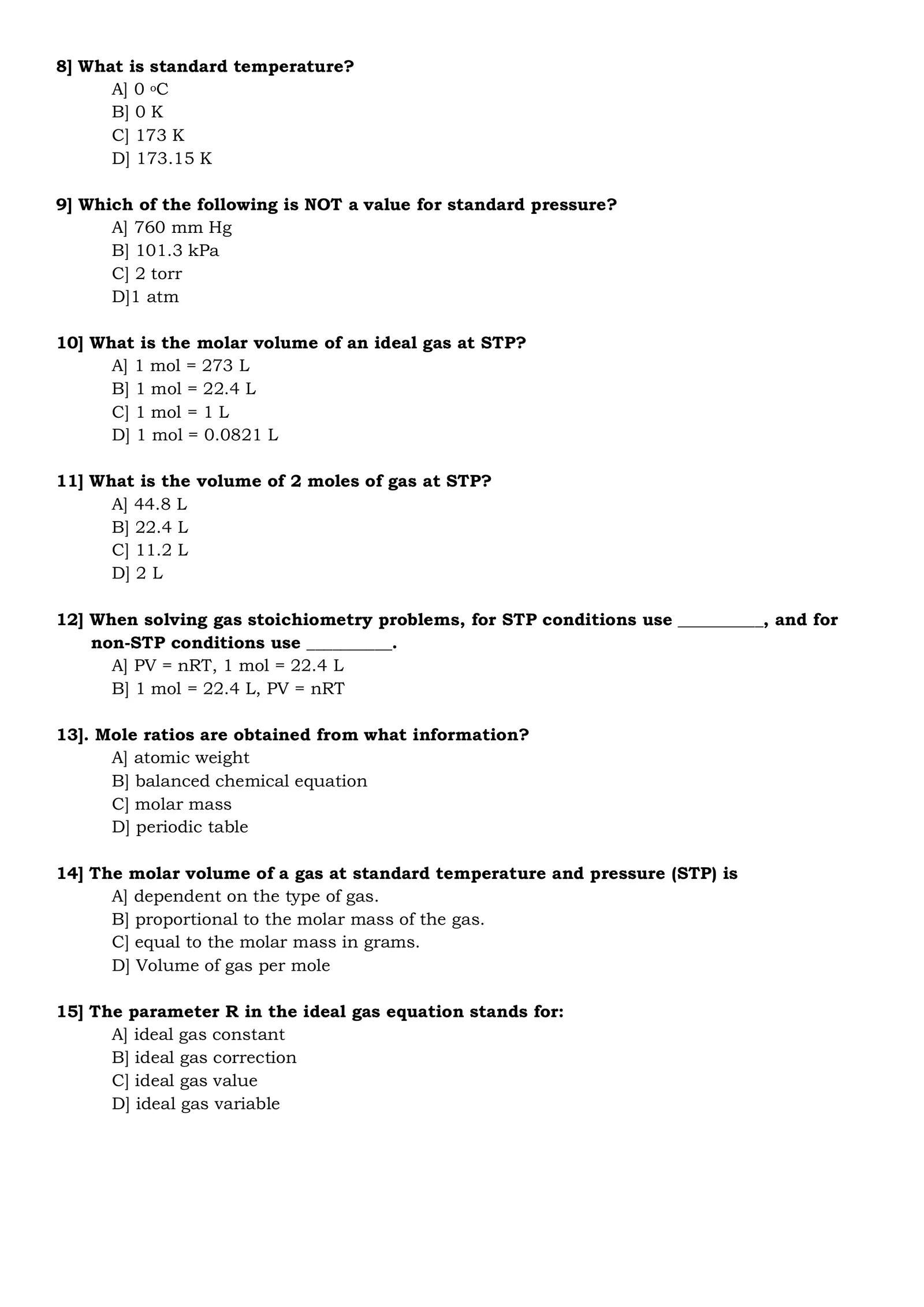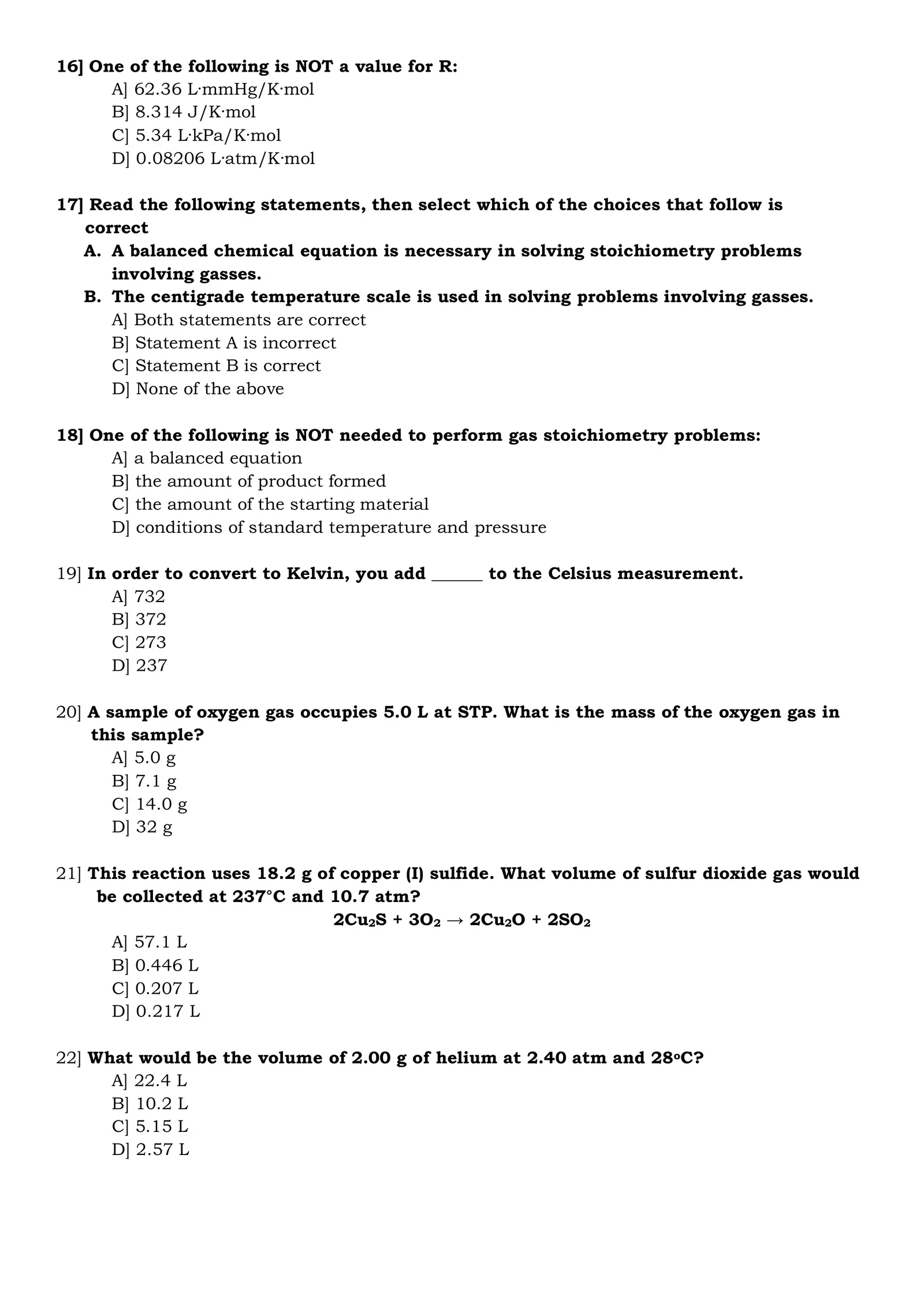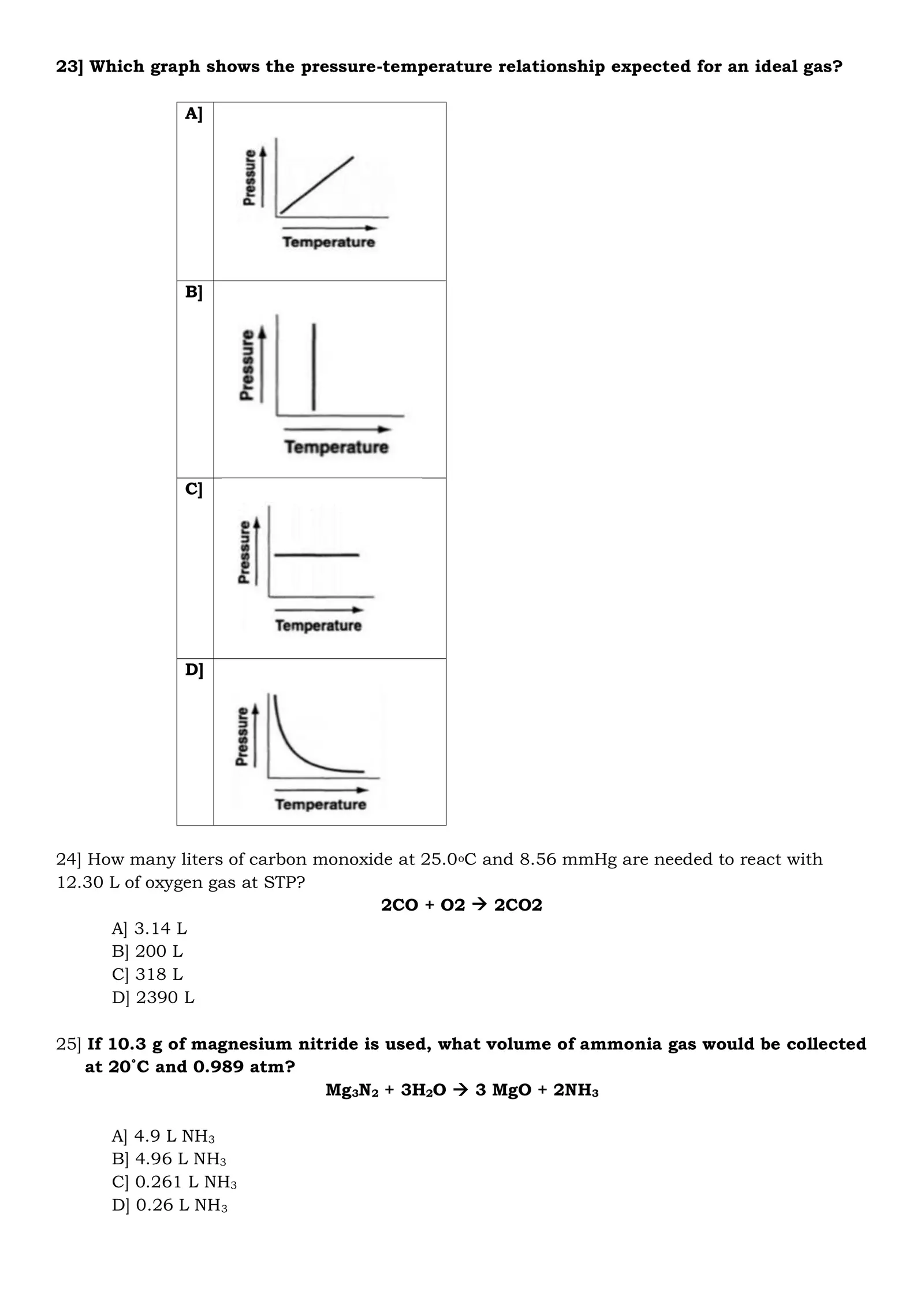# GENCHEM 1 SUMMATIVE TEST 1.4

Created
Best for live in-class or video conferencing lessonsStart teacher-led lessonGENERAL CHEMISTRY 1 SUMMATIVE TEST 1.4 Direction: Select the letter of the correct answer. 1] When solving for gas stoichiometry problems which of the following is correctly matched? A] STP conditions; molar mass B] NON STP conditions; PV=nRT C] STP conditions; PV=nRT D] NON STP conditions; 22.4 moles 2] How do I change from moles to liters? A] divide by 22.4 B] multiply by 22.4 C] multiple by Avo number D] multiply by molar mass 3] How do I move from grams to moles A] divide by molar mass B] multiply by molar mass C] divided by Avo number D] multiply by Avo number 4] How do I change from moles to liters? A] divide by 22.4 B] multiply by 22.4 C] multiple by Avo number D] multiply by molar mass 5] How many atoms are in a mole? A] 1 B] 12.01 C] 22.40 D] 6.022x10^23 6] How many liters are in a mole? A] 1 B] 12.01 C] 22.40 D] 6.022x10^23 7] In the ideal gas law, _____ represents moles of a gas. A] n B] R C] T D] V8] What is standard temperature? A] 0 oC B] 0 K C] 173 K D] 173.15 K 9] Which of the following is NOT a value for standard pressure? A] 760 mm Hg B] 101.3 kPa C] 2 torr D]1 atm 10] What is the molar volume of an ideal gas at STP? A] 1 mol = 273 L B] 1 mol = 22.4 L C] 1 mol = 1 L D] 1 mol = 0.0821 L 11] What is the volume of 2 moles of gas at STP? A] 44.8 L B] 22.4 L C] 11.2 L D] 2 L 12] When solving gas stoichiometry problems, for STP conditions use __________, and for non-STP conditions use __________. A] PV = nRT, 1 mol = 22.4 L B] 1 mol = 22.4 L, PV = nRT 13]. Mole ratios are obtained from what information? A] atomic weight B] balanced chemical equation C] molar mass D] periodic table 14] The molar volume of a gas at standard temperature and pressure (STP) is A] dependent on the type of gas. B] proportional to the molar mass of the gas. C] equal to the molar mass in grams. D] Volume of gas per mole 15] The parameter R in the ideal gas equation stands for: A] ideal gas constant B] ideal gas correction C] ideal gas value D] ideal gas variable16] One of the following is NOT a value for R: A] 62.36 L·mmHg/K·mol B] 8.314 J/K·mol C] 5.34 L·kPa/K·mol D] 0.08206 L·atm/K·mol 17] Read the following statements, then select which of the choices that follow is correct A. A balanced chemical equation is necessary in solving stoichiometry problems involving gasses. B. The centigrade temperature scale is used in solving problems involving gasses. A] Both statements are correct B] Statement A is incorrect C] Statement B is correct D] None of the above 18] One of the following is NOT needed to perform gas stoichiometry problems: A] a balanced equation B] the amount of product formed C] the amount of the starting material D] conditions of standard temperature and pressure 19] In order to convert to Kelvin, you add ______ to the Celsius measurement. A] 732 B] 372 C] 273 D] 237 20] A sample of oxygen gas occupies 5.0 L at STP. What is the mass of the oxygen gas in this sample? A] 5.0 g B] 7.1 g C] 14.0 g D] 32 g 21] This reaction uses 18.2 g of copper (I) sulfide. What volume of sulfur dioxide gas would be collected at 237°C and 10.7 atm? 2Cu2S + 3O2 → 2Cu2O + 2SO2 A] 57.1 L B] 0.446 L C] 0.207 L D] 0.217 L 22] What would be the volume of 2.00 g of helium at 2.40 atm and 28oC? A] 22.4 L B] 10.2 L C] 5.15 L D] 2.57 L23] Which graph shows the pressure-temperature relationship expected for an ideal gas? A] B] C] D] 24] How many liters of carbon monoxide at 25.0oC and 8.56 mmHg are needed to react with 12.30 L of oxygen gas at STP? 2CO + O2 → 2CO2 A] 3.14 L B] 200 L C] 318 L D] 2390 L 25] If 10.3 g of magnesium nitride is used, what volume of ammonia gas would be collected at 20˚C and 0.989 atm? Mg3N2 + 3H2O → 3 MgO + 2NH3 A] 4.9 L NH3 B] 4.96 L NH3 C] 0.261 L NH3 D] 0.26 L NH3